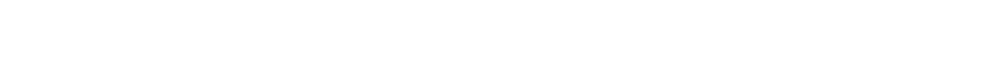#### Revision Notes

##### What are recurring decimals?

A rational number is any number that can be written as an integer (whole number) divided by another integer.  When you write a rational number as a decimal you either get a decimal that stops (eg ¼ = 0.25) or one that recurs (eg ⅓ = 0.333333…).

The recurring part can be written with a dot (or dots) over it instead as in the example below.

##### What do we do with recurring decimals?

Normally, you will be asked to write a recurring decimal as a fraction in its lowest terms.

To do this:

Write out a few decimal places… …and then:

1. Write as f = …
2. Multiply by 10 repeatedly until two lines have the same decimal part
3. Subtract those two lines
4. DIVIDE to get f = … (and cancel if necessary to get fraction in lowest terms)#### Example#### Question#### Answer## Want to aim for a Level 9?

See if you’ve got what it takes. Test yourself with our topic questions.### Author: Simon

After 24 years teaching A Level Further Maths, Maths whizz Simon focused his attention on tutoring. He’s helped thousands of young people make sense of Maths and now he’s helping you too – aren’t you lucky! He’s even worked as an Edexcel examiner, so knows exactly what they’re looking for.